Technical Article

# An Introduction to Rectifier Circuits

June 27, 2016 by Donald Krambeck

## An important application of the diode is one that takes place in the design of the rectifier circuit. Simply put, this circuit converts alternating current (AC) to direct current (DC). This is an essential circuit in AC-to-DC power-supply design.

An important application of the diode is one that takes place in the design of the rectifier circuit. Simply put, this circuit converts alternating current (AC) to direct current (DC). This is an essential circuit in AC-to-DC power-supply design.

### The Rectifier Circuit

In order to power any circuit, a power supply is needed; and if you want to power electronic devices from an AC supply, a rectifier is needed.

Figure 1.1 illustrates a schematic diagram of a DC power supply. There is a 120 V (rms), 60 Hz AC line that feeds the power supply, which delivers a voltage VO to the electronic circuit (load block). VO must be a stable DC voltage to ensure that the electronic circuitry functions correctly.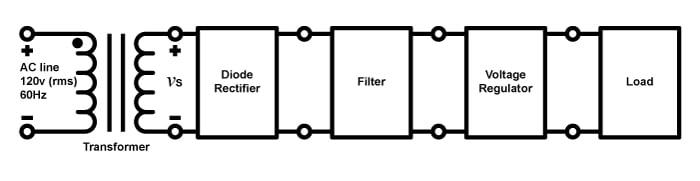##### Figure 1.1

Looking at the diagram, first we see the transformer. This transformer is a step-down transformer that “steps down” the high AC input voltage to a lower AC voltage to be inputted into the rectifier. This transformer consists of two separate coil windings (primary and secondary windings) that have a different number of turns, N1 for the primary and N2 for the secondary. Thus, the AC voltage vS­ can be written as 120(N2/N1) V (rms) and is measured between the two terminals of the secondary winding.

Next, the diode rectifier converts the AC voltage vS to a DC voltage. This voltage will exhibit large variations and thus will not be suitable for electronic circuitry. A filter is used to smooth out these variations.

Even after filtering, though, the voltage will exhibit small variations known as ripple. Consequently, a voltage regulator is used to greatly reduce the ripple and establish a reliable DC supply rail.

### Half-Wave Rectifier Circuit

The half-wave rectifier eliminates the negative portions of the input sinusoid. In Figure 1.2 (A), the half-wave rectifier is illustrated. In this article, we will use the constant voltage drop (CVD) model of a diode owing to its simplicity. From this model, we are provided with

$$v_{0}=0$$  when  $$v_{S}< V_{D}$$

##### Equation 1.1 (A)

$$v_{0}=v_{S}-V_{D}$$  when  $$v_{S}\geq V_{D}$$

##### Equation 1.1 (B)

where VD ≈ 0.7 V. The above equations lead to the transfer characteristic illustrated in Figure 1.2 (B). Figure 1.2 (C) illustrates the voltage output that is provided when the input voltage vS is sinusoidal.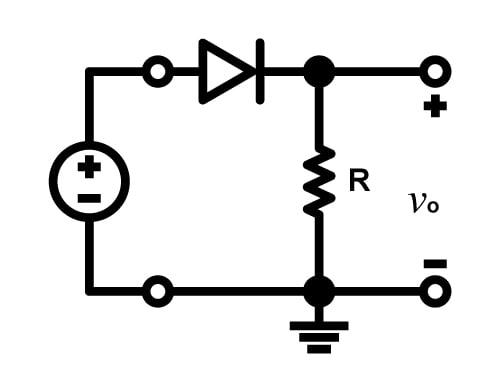##### Figure 1.2 (A) The half-wave rectifier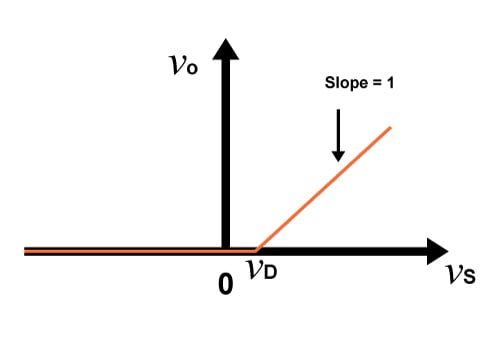##### Figure 1.2 (B) Transfer characteristics of the rectifier circuit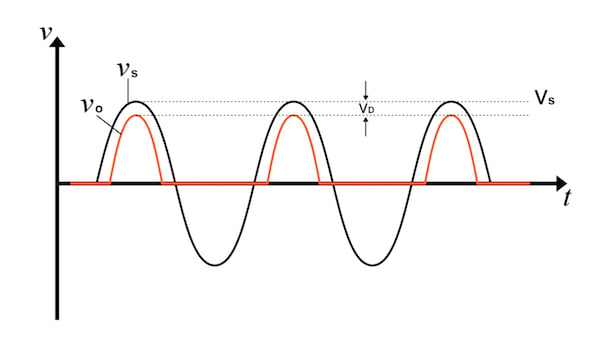##### Figure 1.2 (C) Input and output waveforms

When determining which diodes to use in a rectifier circuit, there are two things to take into consideration: 1) the diode's ability to handle current, which must be chosen based on the largest current that is expected to be conducted by the diode, and 2) the peak inverse voltage (PIV), which is the highest reverse voltage to which the diode will be subjected; the diode must be able to withstand the PIV. Looking at Figure 1.2 (A), we can observe that when the voltage vS is negative, the diode will be cut off and the voltage vO will have a value of zero, leading to a reverse voltage across the diode of magnitude vS. Thus, the PIV is the peak of vS:

PIV = VS

##### Equation 1.2

where VS (with an uppercase V) represents the peak amplitude of the input sinusoid.

One thing worth noting is that the circuit clearly will not operate effectively when the input sinusoid's peak amplitude is not significantly higher than VD. For example, a sinusoidal input with peak amplitude of 200 mV will not be rectified at all because the diode will never "turn on," i.e., it will never conduct significant amounts of current.

### Full-Wave Rectifier Circuit

Unlike the half-wave rectifier, the full-wave rectifier can utilize both the negative and the positive portion of the AC input voltage. In order to achieve a unipolar output, the negative portion of the sinusoidal waveform must be inverted. This can be accomplished by using the circuit shown in Figure 1.3 (A).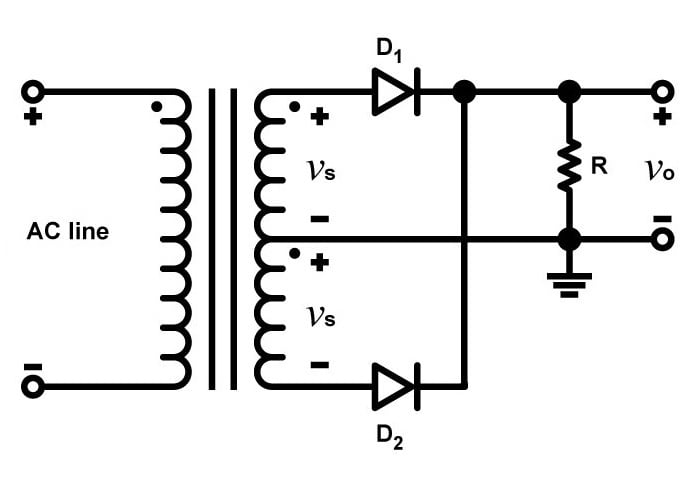##### FIGURE 1.3 (A) Full-wave rectifier circuit; the transformer has a center-tapped secondary winding

In this configuration, the step-down transformer's secondary winding is what is called "center-tapped." A center tap, or CT, is an electrical contact made halfway along the winding. This CT is used to provide two equal voltages, vS, across the two halves of the transformer's secondary winding. When the input voltage is positive, both vS signals will also be positive, and when the input voltage becomes greater than VD, diode D1 will be conducting and diode D2 will be reverse-biased. The current that flows into diode D1 will also flow through resistor R and then back to the CT. The circuit behaves just like the half-wave rectifier during the positive half-cycle of an input sinusoid.

During the negative half-cycle, both vS voltages will be negative. Now, diode D1 is reverse-biased and diode D2 is conducting. The current that flows through D2 will then flow through resistor R and back to the CT.

Thus, current flows during both half-cycles, and furthermore the current through the resistor will always flow in the same direction. The result is a unipolar output voltage, as shown in Figure 1.3 (C).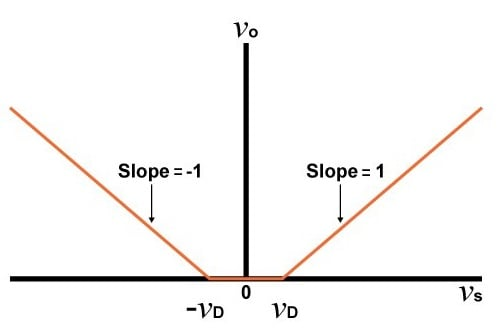##### FIGURE 1.3 (B) Transfer characteristic for the full-wave rectifier

If we consider the circuit's operation during a positive half-cycle, the voltage at the cathode of D2 is (vS - VD) and the voltage at the anode of D2 is -vS. Thus, the PIV is (VS - VD) - (-VS):

PIV = 2VS - VD

##### Equation 1.3

Note that this PIV is roughly double that of the half-wave rectifier.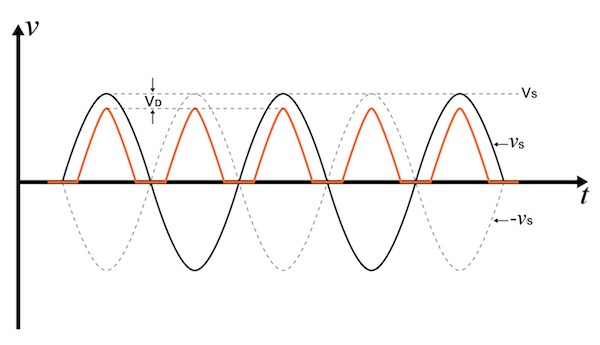### Conclusion

In this article, we discussed the purpose of a rectifier circuit as well as two specific types of rectifiers: the half-wave rectifier and the full-wave rectifier. Rectifiers are essential circuits for power supplies that convert an AC input voltage into a DC voltage supply that can be used to power electronic circuits. We saw that the half-wave rectifier utilizes alternate half-cycles of the input sine wave whereas the full-wave rectifier utilizes both positive and negative half-cycles.

To read more about rectifier circuits, check out the AAC textbook entry here.

1 Comment• V
Vikas Nichit October 26, 2018

Dear Sir,

we are using 3 phase Thyrister type halfwave rectifire to use in coating (CED coating)
Input 420 volt DC output- 75A and 225 voltage.
Rectifire current ratting- 75 A & 225 voltage-
Voltage we can set by prob & Ampere take automatic as per the load
For pure DC output we use to capacitor in series.
we need your help which rating capacitor to be use.
to get better output.

Like.